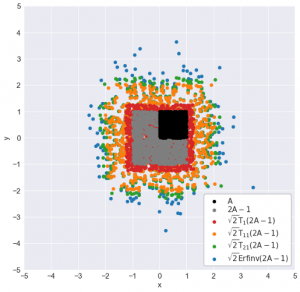# Background

In part 1 of this project, I’ve shown how to generate Gaussian samples using the common technique of inversion sampling:

The bad news: the Gaussian inverse CDF is not well-defined, so we have to approximate that function, and the simple Taylor series was used. However, this only samples accurately near the Gaussian mean, and under-samples more extreme values at both ends of the distribution.A: left-side area (uniformly sampled). Tᵢ: the iᵗʰ-degree Taylor series approximation of the inverse Gaussian CDF: √2 Erfinv(2A-1)

Therefore, in this part of the project, we will investigate a more “direct” sampling method that does not depend on the approximation of the Gaussian inverse CDF. This method is called the Box-Muller transform, after the two mathematicians who invented the method in 1958: the British George E. P. Box and the American Mervin E. Muller.

# How does the Box-Muller transform work?

For this project, my goal is to generate Gaussian samples in two dimensions i.e. generating samples whose x and y coordinates are independent standard normals (Gaussian with zero mean and standard deviation of 1). In part 1, I used the inverse Gaussian CDF to generate separately the x and y coordinates from their respective uniform samples (U₁ and U₂):